# A Truss is Loaded and Supported as Shown.Determine the Following: (1)Identify the Zero Force Members,If Any (2)Find the Forces in Members Ef,Ed and Fc by Method of Joints. - Engineering Mechanics

A truss is loaded and supported as shown.Determine the following:
(1)Identify the zero force members,if any
(2)Find the forces in members EF,ED and FC by method of joints.
(3)Find the forces in members GF,GC and BC by method of sections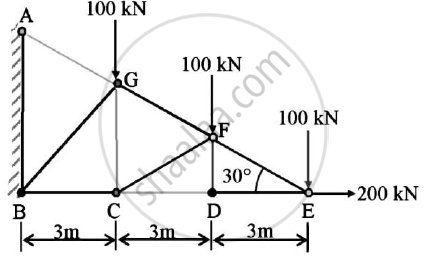#### Solution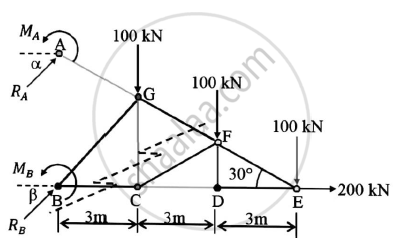By analysis of truss,we can say that DE is zero force member

METHOD OF JOINTS: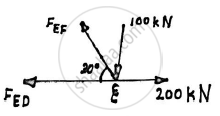Joint E:
Applying the conditions of equilibrium

ΣFY=0
FEFsin30-100=0

FEF=200 kN
Applying the conditions of equilibrium

ΣFX=0
-FEFcos30-FED+200=0
-200cos30+200=FED
FED=26.7949 kN

△FED is congruent to △FCD

∠FCD=∠FED=30°

JOINT F: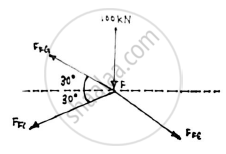Applying the conditions of equilibrium

ΣFY=0
FFGsin30-FFCsin30-FFEsin30-100=0
FFG-FFC-200=200

FFG-FFC=400 ……….(1)

ΣFX=0
-FFGcos30-FFCcos30+FFEcos30=0
Dividing by cos30
FFG +FFC = 200 ………(2)
Solving (1) and (2)
FFG=300 kN
FFC=-100 kN

METHOD OF SECTIONS: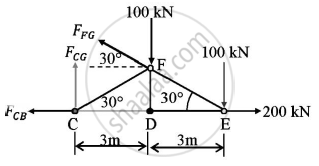In △FED
tan 30 = (FD)/(DE)
DE=3m
FD=sqrt(3) m
Consider the equilibrium of the truss section

ΣMC=0
FFGcos 30 x FD + FFGsin30 x CD -100 x CD -100 X CE = 0
3FFG=900
FFG=300 kN
Applying the conditions of equilibrium

ΣFX=0
-FFGcos30-FcB+200=0

-300cos30+200=FcB
FcB=-59.8076 kN

ΣFY=0
FcG+FFGsin30-100-100=0
FcG=50 kN

 Member of truss Magnitude of force(kN) Nature of force BC 59.8076 Compression GC 50 Tension GF 300 Tension FC 100 Compression ED 26.7949 Tension EF 200 Tension
Concept: Analysis of Plane Trusses by Using Method of Joints
Is there an error in this question or solution?